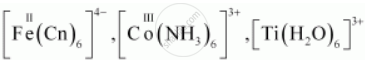Share

# Compare the general characteristics of the first series of the transition metals with those of the second and third series metals in the respective vertical columns. Give special emphasis on the following points - CBSE (Science) Class 12 - Chemistry

ConceptGeneral Properties of the Transition Elements (D-block)

#### Question

Compare the general characteristics of the first series of the transition metals with those of the second and third series metals in the respective vertical columns. Give special emphasis on the following points:

(i) electronic configurations,

(ii) oxidation states,

(iii) ionisation enthalpies, and

(iv) atomic sizes.

#### Solution

1) In the 1st, 2nd and 3rd transition series, the 3d, 4d and 5d orbitals are respectively filled.

We know that elements in the same vertical column generally have similar electronic configurations.

In the first transition series, two elements show unusual electronic configurations:

Cr(24) = 3d^5 4s^1

Cu(29) = 3d^10 4s^1

Similarly, there are exceptions in the second transition series. These are:

Mo(42) = 4d^5 5s^1

Tc(43) = 4d^6 5s^1

Ru(44) = 4d^7 5s^1

Rh(45) = 4d^8 5s^1

Pd(46) = 4d^10 5s^0

Ag(47) = 4d^10 5s^1

There are some exceptions in the third transition series as well. These are:

W(74) = 5d^4 6s^2

Pt(78) = 5d^9 6s^1

Au(79) = 5d^10 6s^1

As a result of these exceptions, it happens many times that the electronic configurations of the elements present in the same group are dissimilar.

(2) In each of the three transition series the number of oxidation states shown by the elements is the maximum in the middle and the minimum at the extreme ends.

However, +2 and +3 oxidation states are quite stable for all elements present in the first transition series. All metals present in the first transition series form stable compounds in the +2 and +3 oxidation states. The stability of the +2 and +3 oxidation states decreases in the second and the third transition series, wherein higher oxidation states are more important.

For exampleare stable complexes, but no such complexes are known for the second and third transition series such as Mo, W, Rh, In. They form complexes in which their oxidation states are high. For example: WCl6, ReF7, RuO4, etc.

(3) In each of the three transition series, the first ionisation enthalpy increases from left to right. However, there are some exceptions. The first ionisation enthalpies of the third transition series are higher than those of the first and second transition series. This occurs due to the poor shielding effect of 4f electrons in the third transition series.

Certain elements in the second transition series have higher first ionisation enthalpies than elements corresponding to the same vertical column in the first transition series. There are also elements in the 2nd transition series whose first ionisation enthalpies are lower than those of the elements corresponding to the same vertical column in the 1st transition series.

(4) Atomic size generally decreases from left to right across a period. Now, among the three transition series, atomic sizes of the elements in the second transition series are greater than those of the elements corresponding to the same vertical column in the first transition series. However, the atomic sizes of the elements in the third transition series are virtually the same as those of the corresponding members in the second transition series. This is due to lanthanoid contraction.

Is there an error in this question or solution?

#### APPEARS IN

NCERT Solution for Chemistry Textbook for Class 12 (2018 to Current)
Chapter 8: The d-block and f-block Elements
Q: 35 | Page no. 236

#### Video TutorialsVIEW ALL 

Solution Compare the general characteristics of the first series of the transition metals with those of the second and third series metals in the respective vertical columns. Give special emphasis on the following points Concept: General Properties of the Transition Elements (D-block).
S